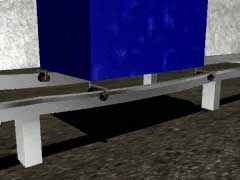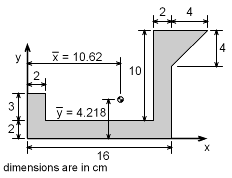Ch 7. Centroid/Distributed Loads/Inertia Multimedia Engineering Statics Centroid: Line Area Vol Centroid: Composite Distributed Loads Area Moment of Inertia
 Chapter 1. Basics 2. Vectors 3. Forces 4. Moments 5. Rigid Bodies 6. Structures 7. Centroids/Inertia 8. Internal Loads 9. Friction 10. Work & Energy Appendix Basic Math Units Sections Search eBooks Dynamics Fluids Math Mechanics Statics Thermodynamics Author(s): Kurt Gramoll ©Kurt GramollSTATICS - CASE STUDY IntroductionLuggage Railway System Once again, the engineers need to study the luggage transport system that was examined previously. This time, they need to understand the effects of the bending on the rail itself by determining the moments of inertia of the cross sectio with respect to an axis through the centroid of the rail. What is known: The centroid is located at x = 10.62 cm and y = 4.218 cm (calculated previously). The dimensions of the cross section are as shown. QuestionCentroid and Dimension Diagram What are the moments of inertia for the entire cross section with respect to the centroidal axis? Approach Determine the moments of inertia for each individual part with respect to its centroid. Use the parallel axis theorem and the method of composite parts to determine the moments of inertia for the centroidal axis of the cross section.

Practice Homework and Test problems now available in the 'Eng Statics' mobile app
Includes over 500 problems with complete detailed solutions.
Available now at the Google Play Store and Apple App Store.